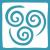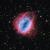# Polyhedron## Truncated Icosidodecahedron

The truncated icosidodecahedron is made up of 30 square faces, 20 regular hexagonal faces, 12 regular decagonal faces, 120 vertices and 180 edges. The dihedral angles for this Archimedean solid are as follows:

• between a hexagonal face and a decagonal face: 142.62 degrees (6-10)

• between a square face and a decagonal face: 148.28 degrees (4-10)

• between a square face and a hexagonal face: 159.095 degrees (4-6)## Tetrahedron

The tetrahedron has the smallest number of faces in the five Platonic Solids, having only 4 faces. And in fact, four faces are the minimium requirement for a polyhedron. Other features of tetrahedron includes:

• Each face is an equilaterial triangle
• Each vertex is the meeting of 3 faces## Snub dodecahedron

The Snub dodecahedron has 92 faces (12 pentagons, 80 equilateral triangles), 150 edges,  and 60 vertices.